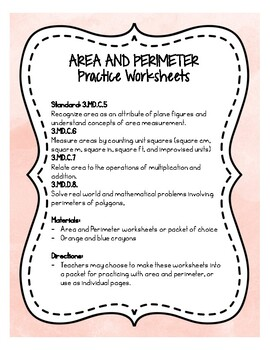# Area and Perimeter: Practice WorksheetsSubject
Resource Type
File Type

PDF

(1 MB|11 pages)
Product Rating
Standards
Also included in:
1. Games for math centers, practice worksheets, interactive activities, and enrichment opportunities are provided in this bundle. The activities follow third grade math standards, but could be used across grade levels.
\$12.50
\$11.25
Save \$1.25
• Product Description
• StandardsNEW

There are 5 different worksheets included in this resource. There is a cover page if you choose to make the worksheets into a packet format for student practice. Answer keys are provided for each worksheet.

Worksheet 1: Rectangles with side measurements are provided, and students must determine the area and perimeter of each.

Worksheet 2: Rectangles are on a grid, and students must determine the area and perimeter of each.

Worksheet 3: Irregular shapes are provided on a grid, and students must count the area and perimeter of each.

Worksheet 4: Rectangular shapes with side lengths are provided, students must determine the area and perimeter, and then color in figures that have the same area and a different perimeter.

Worksheet 5: Rectangular shapes with side lengths are provided, students must determine the area and perimeter, and then color in figures that have the same perimeter and a different area.

Recognize area as additive. Find areas of rectilinear figures by decomposing them into non-overlapping rectangles and adding the areas of the non-overlapping parts, applying this technique to solve real world problems.
Use tiling to show in a concrete case that the area of a rectangle with whole-number side lengths 𝘢 and 𝘣 + 𝘤 is the sum of 𝘢 × 𝘣 and 𝘢 × 𝘤. Use area models to represent the distributive property in mathematical reasoning.
Multiply side lengths to find areas of rectangles with whole-number side lengths in the context of solving real world and mathematical problems, and represent whole-number products as rectangular areas in mathematical reasoning.
Find the area of a rectangle with whole-number side lengths by tiling it, and show that the area is the same as would be found by multiplying the side lengths.
Relate area to the operations of multiplication and addition.
Total Pages
11 pages
N/A
Teaching Duration
N/A
Report this Resource to TpT
Reported resources will be reviewed by our team. Report this resource to let us know if this resource violates TpT’s content guidelines.